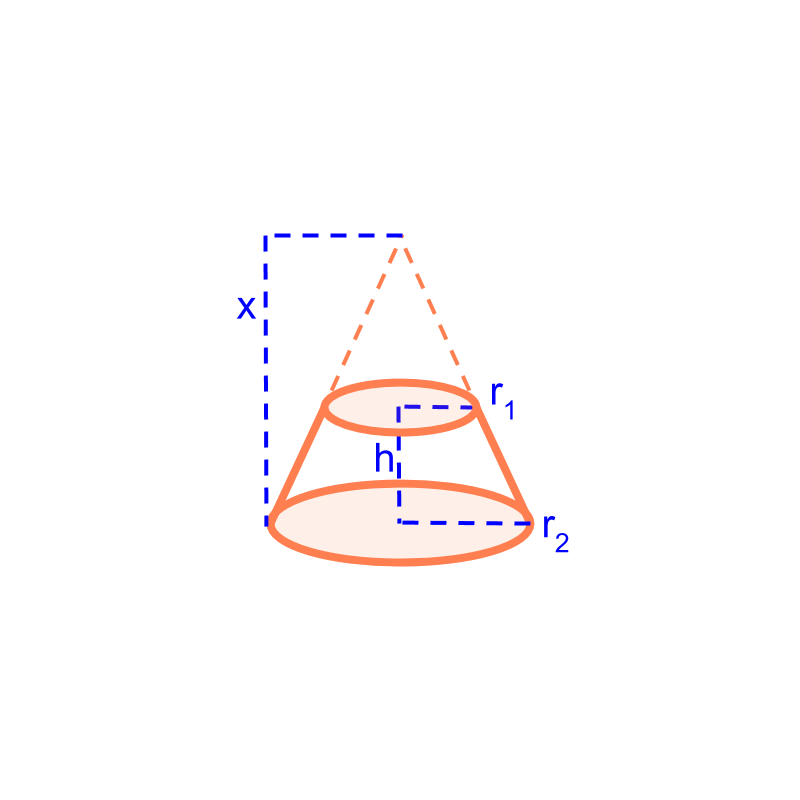maths > mensuration-high

Surface Area and Volume of Frustum of a Cone

what you'll learn...

Overview

Surface Area and Volume of a frustum:The frustum is visualized as part of a cone and the height of the cone is worked out from the given height and radius of frustum.

Surface Area of frustum is the difference between surface area of larger cone and smaller cone.
$=\pi \left({r}_{1}+{r}_{2}\right)\sqrt{{h}^{2}+{\left({r}_{2}-{r}_{1}\right)}^{2}}$$= \pi \left({r}_{1} + {r}_{2}\right) \sqrt{{h}^{2} + {\left({r}_{2} - {r}_{1}\right)}^{2}}$

Volume of frustum is the difference between volume of larger cone and smaller cone.
$=\frac{1}{3}\pi h\left({r}_{1}^{2}+{r}_{2}^{2}+{r}_{1}{r}_{2}\right)$$= \frac{1}{3} \pi h \left({r}_{1}^{2} + {r}_{2}^{2} + {r}_{1} {r}_{2}\right)$

frustum

The curved surface area of a cone of height $h$$h$ and radius $r$$r$ is "$\pi r\left(r+l\right)$$\pi r \left(r + l\right)$", where $l$$l$ is the slant length $l=\sqrt{{r}^{2}+{h}^{2}}$$l = \sqrt{{r}^{2} + {h}^{2}}$

surface areaConsider the frustum with height $h$$h$, radius ${r}_{1}$${r}_{1}$ and ${r}_{2}$${r}_{2}$.

The figure illustrates the larger cone from which the frustum is formed. The part of the cone sliced is the smaller cone. To find the curved surface area of the frustum, Subtract the surface area of the smaller cone from the surface area of the larger cone. It is noted that the height of the larger cone can be found using the height and the two radius of frustum.

The parameters of the frustum are $h$$h$, ${r}_{1}$${r}_{1}$ and ${r}_{2}$${r}_{2}$. We have to find the height of larger cone $x$$x$.

It is noted that the $x$$x$ and $h$$h$ form a side of two similar triangles. The triangles have ${r}_{1}$${r}_{1}$ and ${r}_{2}$${r}_{2}$ as the otherside.

Using the property of similar triangles, it is derived as $x=h{r}_{2}/\left({r}_{2}-{r}_{1}\right)$$x = h {r}_{2} / \left({r}_{2} - {r}_{1}\right)$

Curved surface area of frustum $=$$=$curved surface area of larger cone - curved surface area of smaller cone $=\pi {r}_{2}\left({r}_{2}+\sqrt{{r}_{2}^{2}+{x}^{2}}\right)$$= \pi {r}_{2} \left({r}_{2} + \sqrt{{r}_{2}^{2} + {x}^{2}}\right)$$-\pi {r}_{1}\left({r}_{1}+\sqrt{{r}_{1}^{2}+{\left(x-h\right)}^{2}}\right)$$- \pi {r}_{1} \left({r}_{1} + \sqrt{{r}_{1}^{2} + {\left(x - h\right)}^{2}}\right)$ $=\pi \left({r}_{1}+{r}_{2}\right)\sqrt{{h}^{2}+{\left({r}_{2}-{r}_{1}\right)}^{2}}$$= \pi \left({r}_{1} + {r}_{2}\right) \sqrt{{h}^{2} + {\left({r}_{2} - {r}_{1}\right)}^{2}}$

volume

The volume of a cone of height $h$$h$ and radius $r$$r$ is "$\frac{1}{3}\pi {r}^{2}h$$\frac{1}{3} \pi {r}^{2} h$"

Consider the frustum with height $h$$h$ and radius ${r}_{1}$${r}_{1}$ and ${r}_{2}$${r}_{2}$.

The figure illustrates the larger cone from which the frustum is formed. The part of the cone sliced is the smaller cone.

To find the volume of the frustum, Subtract the volume of the smaller cone from the volume of the larger cone.

The parameters of the frustum are $h$$h$, ${r}_{1}$${r}_{1}$ and ${r}_{2}$${r}_{2}$. We have derived the height of larger cone $x=h{r}_{2}/\left({r}_{2}-{r}_{1}\right)$$x = h {r}_{2} / \left({r}_{2} - {r}_{1}\right)$

Volume of frustum

$=$$=$volume of larger cone - volume of smaller cone

$=\frac{1}{3}\pi {r}_{2}^{2}h{r}_{2}/\left({r}_{2}-{r}_{1}\right)$$= \frac{1}{3} \pi {r}_{2}^{2} h {r}_{2} / \left({r}_{2} - {r}_{1}\right)$$-\frac{1}{3}\pi {r}_{1}^{2}\left(-h+h{r}_{2}/\left({r}_{2}-{r}_{1}\right)\right)$$- \frac{1}{3} \pi {r}_{1}^{2} \left(- h + h {r}_{2} / \left({r}_{2} - {r}_{1}\right)\right)$

$=\frac{1}{3}\pi h\left({r}_{1}^{2}+{r}_{2}^{2}+{r}_{1}{r}_{2}\right)$$= \frac{1}{3} \pi h \left({r}_{1}^{2} + {r}_{2}^{2} + {r}_{1} {r}_{2}\right)$

What is the volume of a frustum with both top and bottom radius $r$$r$ and height $h$$h$?

It is a cylinder, so volume $=\pi {r}^{2}h$$= \pi {r}^{2} h$

As a frustum, the volume $=\frac{1}{3}\pi h\left({r}^{2}+{r}^{2}+r×r\right)$$= \frac{1}{3} \pi h \left({r}^{2} + {r}^{2} + r \times r\right)$, which equals the volume of cylinder.

summary

Surface Area and Volume of a frustum:The frustum is visualized as part of a cone and the height of the cone is worked out from the given height and radius of frustum.

Surface Area of frustum is the difference between surface area of larger cone and smaller cone.
$=\pi \left({r}_{1}+{r}_{2}\right)\sqrt{{h}^{2}+{\left({r}_{2}-{r}_{1}\right)}^{2}}$$= \pi \left({r}_{1} + {r}_{2}\right) \sqrt{{h}^{2} + {\left({r}_{2} - {r}_{1}\right)}^{2}}$

Volume of frustum is the difference between volume of larger cone and smaller cone.
$=\frac{1}{3}\pi h\left({r}_{1}^{2}+{r}_{2}^{2}+{r}_{1}{r}_{2}\right)$$= \frac{1}{3} \pi h \left({r}_{1}^{2} + {r}_{2}^{2} + {r}_{1} {r}_{2}\right)$

Outline